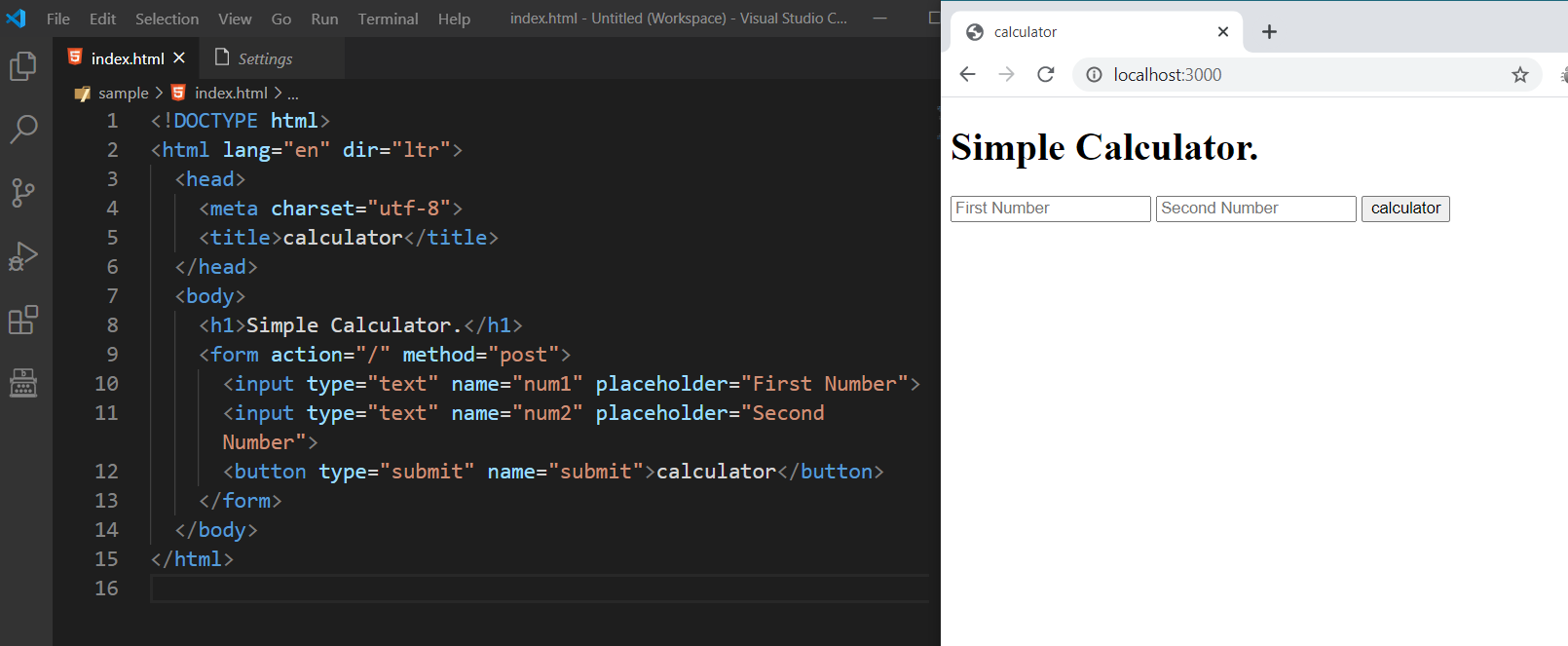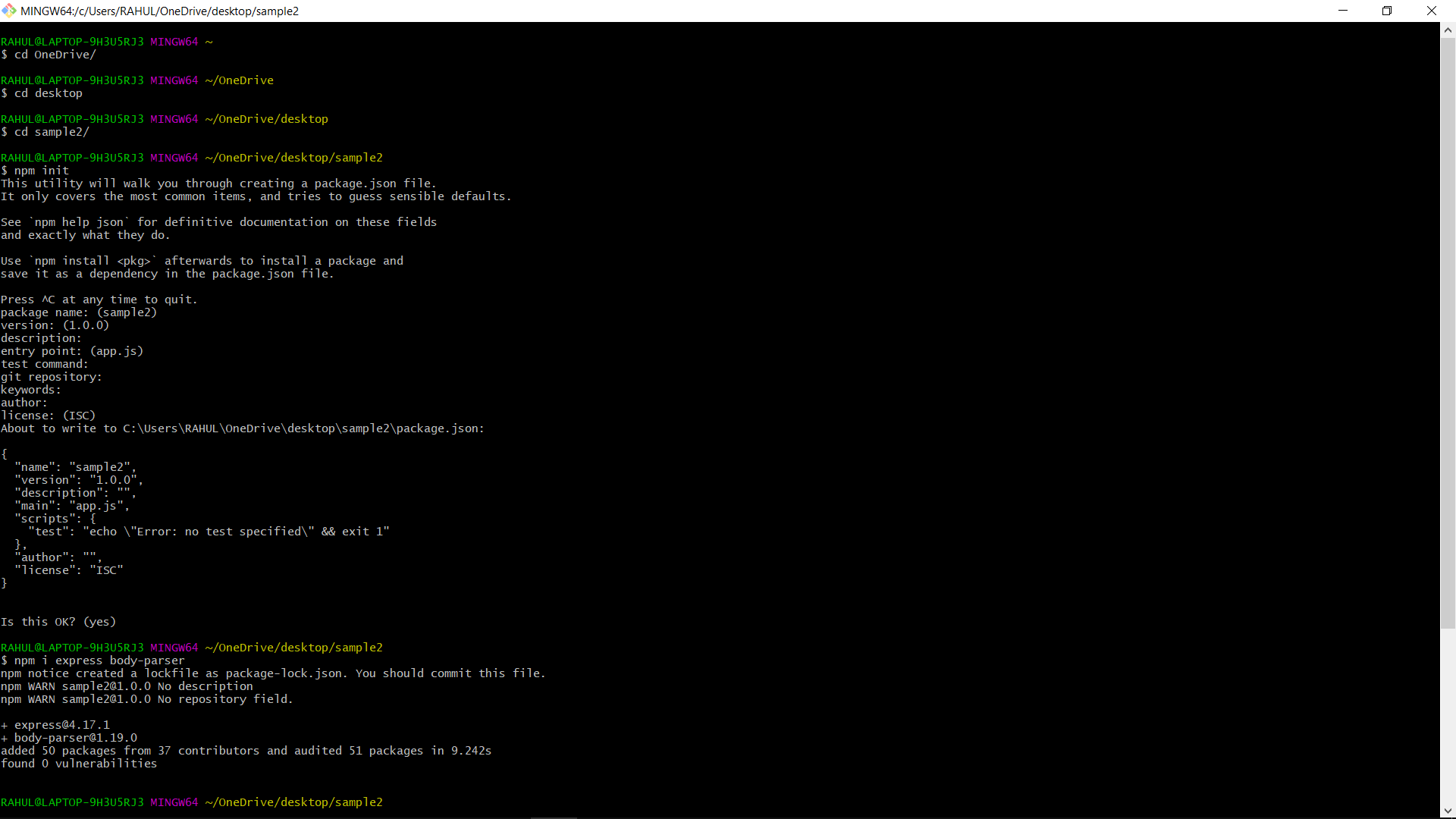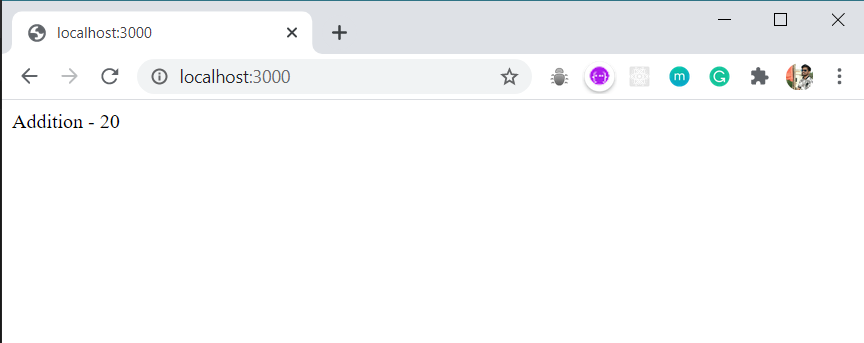Open in App
Not now

# How HTTP POST request work in Node.js?

• Difficulty Level : Hard
• Last Updated : 07 Oct, 2021

POST is a request method supported by HTTP used by the World Wide Web. The HTTP POST method sends data to the server. The type of the body of the request is indicated by the Content-Type header. We use Express.js in order to create a server and to make requests (GET, POST, etc).

`npm i express`

Note: The npm in the above commands stands for node package manager, a place from where we install all the dependencies.

So in order to use Express to address POST requests on our server, we use the app.post method and then we specify the route, and we have a callback.

```app.post(route, function(req, res){
//this is a callback function
})```

Note: If you are going to make GET, POST request frequently in NodeJS, then use Postman , Simplify each step of building an API.

In this syntax, the route is where you have to post your data that is fetched from the HTML. For fetching data you can use bodyparser package.

Web Server: Create app.js in the root folder. Create your server as shown in the below example.

## javascript

 `const express = require(``"express"``); ` `const app = express(); ` ` `  `// Define routes here ... ` ` `  `app.listen(3000, ``function``(){ ` `  ``console.log(``"server is running on port 3000"``); ` `})`

Handle Post Request: Here you will learn how to handle HTTP POST request and get data from the submitted form.

Create index.html in the root folder of your application and write following HTML code in it.

Filename: index.html

## html

 ` ` `<``html` `lang``=``"en"` `dir``=``"ltr"``> ` ` `  `<``head``> ` `    ``<``meta` `charset``=``"utf-8"``> ` `    ``<``title``>Calculator ` ` ` ` `  `<``body``> ` `    ``<``h1``>Simple Calculator. ` `    ``<``form` `action``=``"/"` `method``=``"post"``> ` `        ``<``input` `type``=``"text"` `name``=``"num1"`  `            ``placeholder``=``"First Number"``> ` `        ``<``input` `type``=``"text"` `name``=``"num2"`  `            ``placeholder``=``"Second Number"``> ` `             `  `        ``<``button` `type``=``"submit"` `name``=``"submit"``> ` `            ``calculator ` `        `` ` `    `` ` ` ` ` `  ` `

Output:Handle POST Route in Express.js:
Filename: app.js

## javascript

 `const express = require(``"express"``); ` `const bodyParser = require(``"body-parser"``) ` ` `  `// New app using express module ` `const app = express(); ` `app.use(bodyParser.urlencoded({ ` `    ``extended:``true` `})); ` ` `  `app.get(``"/"``, ``function``(req, res) { ` `  ``res.sendFile(__dirname + ``"/index.html"``); ` `}); ` ` `  `app.post(``"/"``, ``function``(req, res) { ` `  ``var` `num1 = Number(req.body.num1); ` `  ``var` `num2 = Number(req.body.num2); ` `   `  `  ``var` `result = num1 + num2 ; ` `   `  `  ``res.send(``"Addition - "` `+ result); ` `}); ` ` `  `app.listen(3000, ``function``(){ ` `  ``console.log(``"server is running on port 3000"``); ` `}) `

Steps To Run:

• npm init
• npm install express
• npm install body-parser
• node app.js
• go to http://localhost:3000 in your browser.Output:My Personal Notes arrow_drop_up
Related Articles